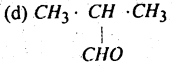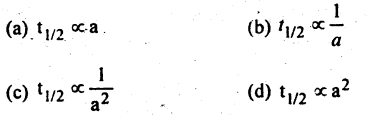# Bihar Board 12th Chemistry Objective Important Questions Part 5 in English

BSEB Bihar Board 12th Chemistry Important Questions Objective Type Part 5 are the best resource for students which helps in revision.

## Bihar Board 12th Chemistry Objective Important Questions Part 5 in English

Question 1.
Chloral is:
(a) CCl3CHO
(b) CCl3CH3
(c) CCl3COCH3
(d) CCl3CH2OH
(a) CCl3CHO

Question 2.
Amongst the following the most reactive alkyl halide is :
(a) C2H5Br
(b) C2H5I
(c) C2H5Cl
(d) All are same
(a) C2H5Br

Question 3.
Amongst the following which one is the most reactive?
(a) CH3CH2I
(b) C2H5Br
(c) (CH3)3Cl
(d) All are same
(c) (CH3)3Cl

Question 4.
Ethyl iodide on treatment with moist silver oxide gives :
(a) acetic acid
(b) ethyl alcohol
(c) acetaldehyde
(d)none
(b) ethyl alcohol

Question 5.
Amongst the following, the one having the highest boiling point is :
(a) C2H5Cl
(b) C2H5Br
(c) C2H5I
(d) CH3Cl
(c) C2H5I

Question 6.
Which one of the following will give ethyl alcohol when heated with moist silver oxide:
(a) CH3I
(b) C2H5Br
(c) (CH3)2CHI
(d) CH3-CH2-CH2I
(b) C2H5Br

Question 7.
Amongst the following which one has the highest boiling point?
(a) ethyl alcohol
(b) n-propyl alcohol
(c) n-butyl alcohol
(d) methyl alcohol
(c) n-butyl alcohol

Question 8.
Which one is the most acidic?
(a) CH3OH
(b) C2H5OH
(c) CH3·CH(OH)·CH3
(d) CH3·CH2·CH2OH
(a) CH3OH

Question 9.
Ethyl alcohol, when oxidised with acidic K2Cr2O7 solution, gives:
(a) CH3·COOH
(b) HCOOH
(c) CH3·CO·CH3
(d) None
(a) CH3·COOH

Question 10.
Which one of the following will give an aldehyde on oxidation with chromic anhydride in glacial acetic acid?
(a) CH2COOH
(b) CH3CH(OH)CH3
(c) CH3 CH2OH
(d) None
(c) CH3 CH2OH

Question 11.
Which one of the following will give a ketone on oxidation with acidic K2Cr2O7 ?
(a) CH3 CH2OH
(b) CH3CH (OH)CH3
(c) CH3OH
(d) None
(b) CH3CH (OH)CH3

Question 12.
Which one of the following reduces Fehling’s solution?
(a) CH3COOH
(b) CH3COCH3
(c) HCHO
(d) CH3OH
(c) HCHO

Question 13.
Which one of the following undergoes Cannizzaro reaction?
(a) HCHO
(b) CH3 CHO
(c) CH3COCH3(a) HCHO

Question 14.
Dry distillation of calcium acetate will give :
(a) CH3 COOH
(b) CH3COCH3
(c) CH3 CHO
(d) CH3 · CH3
(b) CH3COCH3

Question 15.
Which will turn blue litmus red?
(a) HCOOH
(b) CH3CHO
(C) CH3COCH3
(d) None
(a) HCOOH

Question 16.
When a mixture of calcium acetate and calcium formate is heated. it yields:
(a) acetone
(b) formic acid
(e) acetic acid
(d) acetaldehyde
(d) acetaldehyde

Question 17.
Carboxylic acids do not give the characteristic properties of:
(a) >C = O group
(b) -COOH group
(c) Alkyl group
(d) none of these
(a) >C = O group

Question 18.
Which one is a phenol?
(a) C6H5CH2OH
(b) C6H5OH
(c) CH3CH2OH
(d) None
(b) C6H5OH

Question 19.
Which is the strongest acid
(a) p-phenol
(b) m-phenol
(c) o-phenol
(d) phenol
(a) p-phenol

Question 20.
Which of the following turns litmus red?
(a) CH3CH2OH
(b) C6H5CHO
(c) C6H5OH
(d) None
(c) C6H5OH

Question 21.
Phenols do not react with :
(a) NaOH
(b) KOH
(c) NaHCO3
(d) None
(c) NaHCO3

Question 22.
Amongst the following the most acidic oxide is:
(a) Sb2O5
(b) P2O5
(c) N2O5
(d) AS2O5
(c) N2O5

Question 23.
The cause of variable valency-among transition dementias:
(a) They all exist in more than one oxidation state
(b) They all form complex compounds
(c) the valence electrons of them are found in two different subshells
(d) they all have paired electrons
(c) the valence electrons of them are found in two different subshells

Question 24.
Which one is not a transition element?
(a) Co
(b) V
(c) Ge
(d) Rh
(c) Ge

Question 25.
Froth floatation process is used :
(a) for calcination of ores
(b) for roasting of ores
(c) for concentration of ores
(d) for smelting of ores
(c) for concentration of ores

Question 26.
Sulphide ores are concentrated by :
(a) gravity separation
(b) electromagnetic separation
(c) froth floatation process
(d) None of these
(c) froth floatation process

Question 27.
Froth floatation process is used for the concentration of:
(a) sulphide ores
(b) carbonate ores
(c) oxide ores
(d) sulphate ores
(a) sulphide ores

Question 28.
Which of the following ores is concentrated by froth floatation process:
(a) Bauxite
(b) cassiterite
(c) galena
(d) siderite
(c) galena

Question 29.
When one Faraday of Electric current is passed. The Mass deposited is equal to :
(a) One gram equivalent
(b) One gram Mole
(c) Electro-chemical equivalent
(d) Half gram equivalent
(a) One gram equivalent

Question 30.
Amongst the following the most acidic oxide is :
(a) N2O3
(b) N2O5
(c) N2O4
(d) All are same
(b) N2O5

Question 31.
Thermally most stable oxide is :
(a ) P2O3
(b) As2O3
(c) Sb2O3
(d) N2O3
(d) N2O3

Question 32.
Which is the most stable:
(a) N2O3
(b) N2O5
(c) N2O4
(d) All are same
(a) N2O3

Question 33.
Which one is the most basic?
(a) NH3
(b) AsH3
(c) SbH3
(d) PH3
(a) NH3

Question 34.
Which one is the most stable?
(a) PH3
(b) NH3
(c) SbH3
(d) AsH3
(b) NH3

Question 35.
Which is stronger reducing agent?
(a) PH3
(b) NH3
(c) SbH3
(d) AsH3
(c) SbH3

Question 36.
Which of the folIowingeOmpounds is least acidic?
(a) H2Se
(b) H2O
(c) H2S
(d) H2Te
(b) H2O

Question 37.
Which of the following compounds is most stable?
(a) H2O
(b) H2Te
(c) H2S
(d) H2Se
(a) H2O

Question 38.
Which of the following is the strongest reducing agent?
(a) H2O
(b) H2Se
(c) H2Te
(d) H2S
(c) H2Te

Question 39.
Which of the following aqueous solution should have highest boiling point:
(a) 1.0 M NaOH
(b) 1.0 M Na2SO4
(c) 1.0 M NH4NO3
(d) 1.0 M KNO3
(b) 1.0 M Na2SO4

Question 40.
A solution has an osmotic pressure of 0.0821 atm at 300K. Its concentration would be:
(a) 0.66 M
(b) 0.32 M
(c) 0.066 M
(d) 0.0033 M
(d) 0.0033 M

Question 41.
Azeotropic mixture of HCl and H2O has :
(a) 48% HCl
(b) 22.2% HCl
(c) 36% HCl
(d) 20.2% HCl
(d) 20.2% HCl

Question 42.
For a zero order reaction :(a) t1/2 ∝ a

Question 43.
The rate at which a substance reacts depends upon its:
(a) Atomic mass
(b) Equivalent mass
(c) Molecular mass
(d) Active mass
(d) Active mass

Question 44.
Which of the following is Tribasic :
(a) H3PO2
(b) H3PO3
(c) H4P2O7
(d) H3PO4
(d) H3PO4

Question 45.
Froth floatation process is based on :
(a) specific gravity of ore particles
(b) magnetic properties of the ore particles
(c) electrical properties of the ore particles
(d) wetting properties of the ore particles